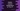# 2 ways in Python to convert temperature in Celsius to Fahrenheit## Python program to convert temperature in Celsius to Fahrenheit:

This post will show you how to write a python program that converts the temperature in Celsius to Fahrenheit. It will take the temperature in celsius as input from the user, convert it to Fahrenheit and print it out.

Let’s learn the algorithm first before we start writing the program.

### Algorithm to convert temperature in Celsius to Fahrenheit:

We have to use the below formula to convert a temperature value in Celsius to Fahrenheit:

``Fahrenheit = (Celsius * 1.8) + 32``

The program will use the below algorithm:

• Take the Celsius value as input from the user.
• Use the above formula to convert the Celsius value to Fahrenheit.
• Print the Fahrenheit value.

That’s it.

### Method 1: Python program to convert Celsius to Fahrenheit:

Below is the complete program that uses the above algorithm to do the Celsius-Fahrenheit conversion:

``````celsius = float(input("Enter the temperature in Celsius: "))
fahrenheit = (celsius * 1.8) + 32

print(f'{celsius}°C = {fahrenheit}°F')``````

Here,

• We are using the input method to read the user input Celsius value. This is a string value. So, we converted it to float and assigned it to the variable celsius.
• The Fahrenheit value is calculated with the above formula and assigned to the variable fahrenheit.
• The last line is printing the converted result.

If you run this program, it will print output as below:

``````Enter the temperature in Celsius: 221
221.0°C = 429.8°F

Enter the temperature in Celsius: 122
122.0°C = 251.6°F``````

### Method 2: Python program for Celsius to Fahrenheit conversion by using a separate method:

We can place this code in a separate method to do the conversion. This method will convert the Celsius value to Fahrenheit and return it.

``````def to_fahrenheit(celsius):
return (celsius * 1.8) + 32

celsius = float(input("Enter the temperature in Celsius: "))
print(f'{celsius}°C = {to_fahrenheit(celsius)}°F')``````

We can call this method from anywhere we want and it will give the same result.

``````Enter the temperature in Celsius: 121
121.0°C = 249.8°F

Enter the temperature in Celsius: 221
221.0°C = 429.8°F``````